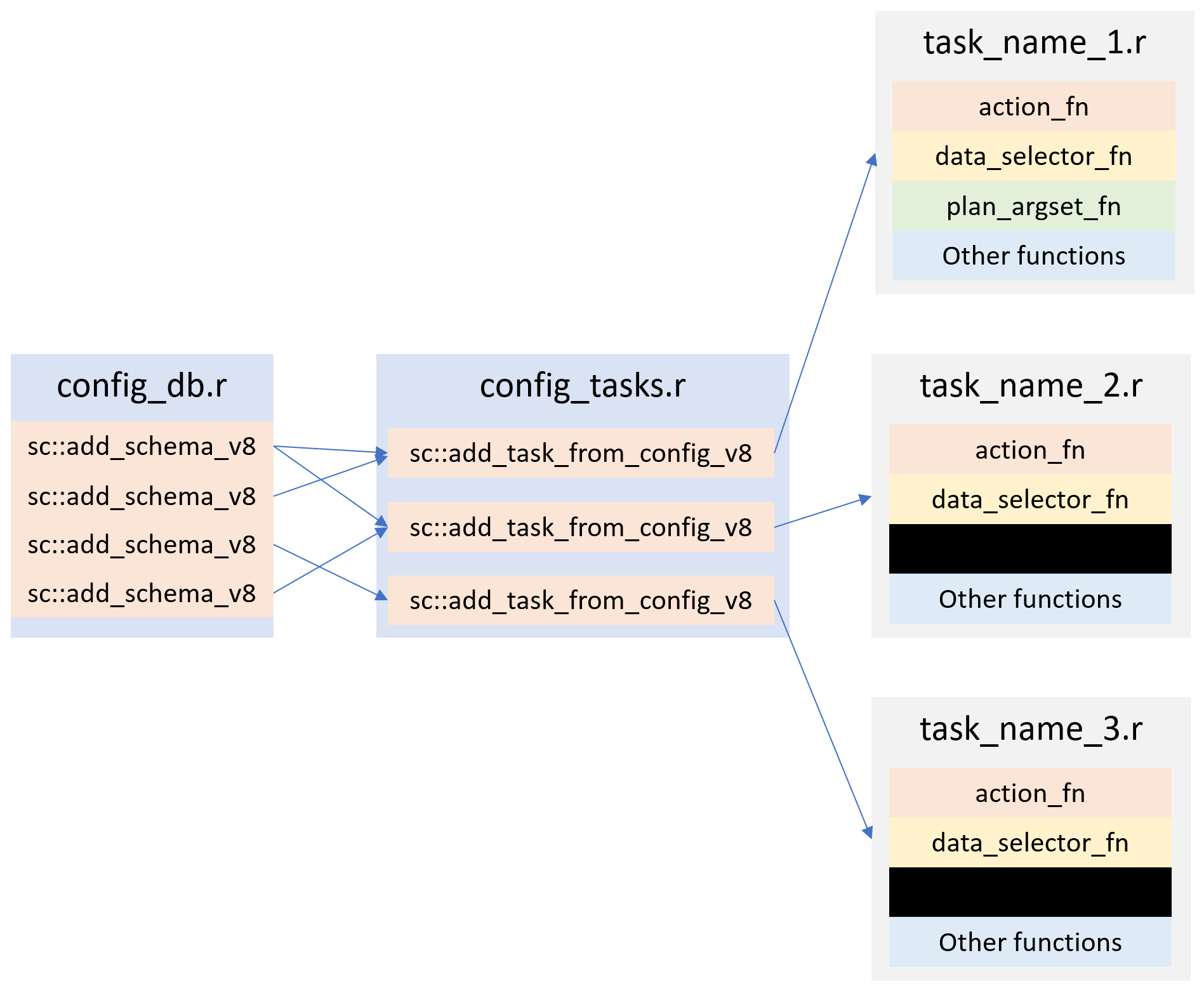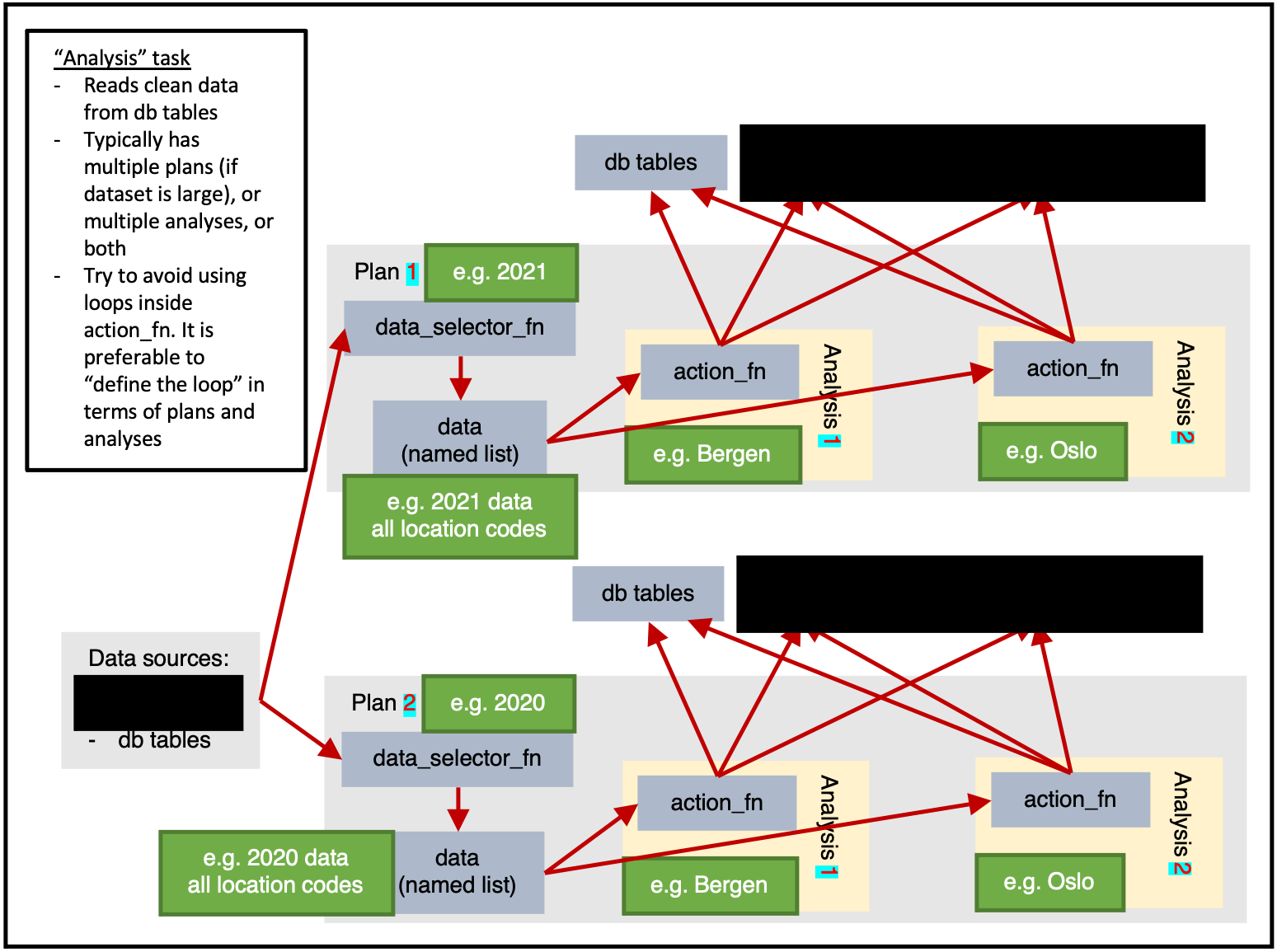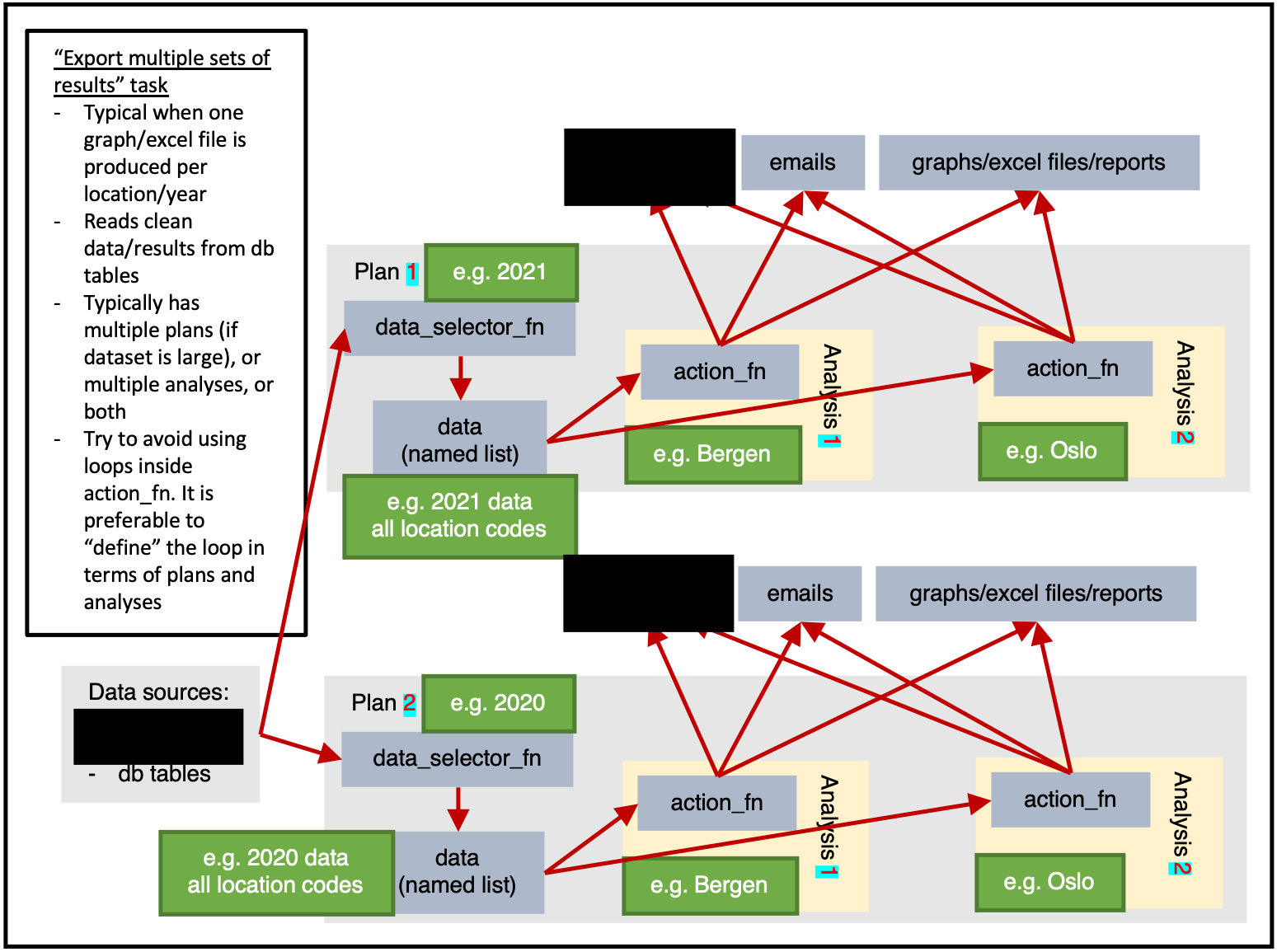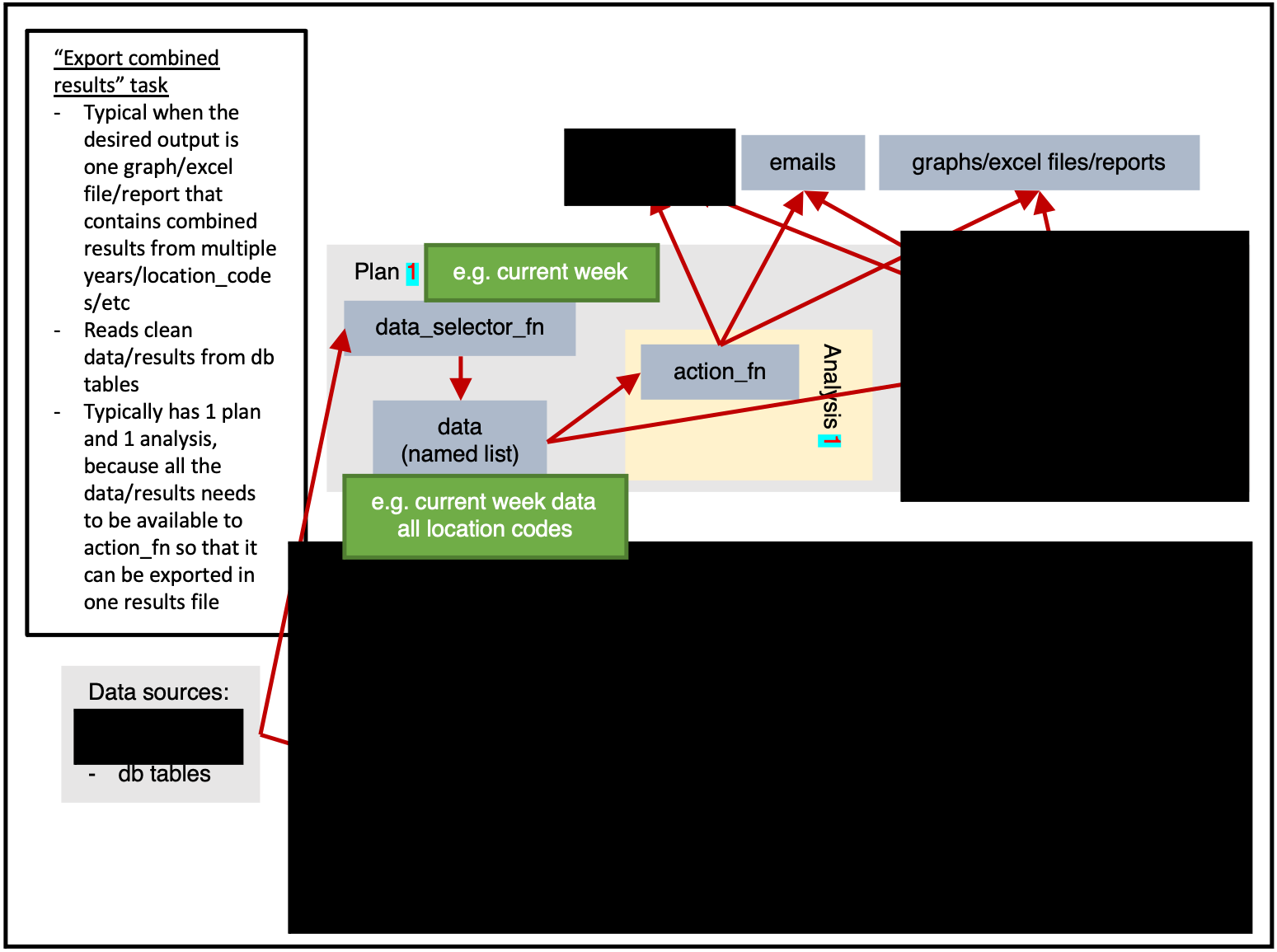## Definitions

Object Description
argset A named list containing arguments.
plnr analysis These are the fundamental units that are scheduled in plnr:
• 1 argset
• 1 function that takes two (or more) arguments:
• data (named list)
• argset (named list)
• … (optional arguments)
data_selector_fn A function that takes two arguments:
• argset (named list)
• tables (named list)
This function provides a named list to be used as the data argument to action_fn
action_fn A function that takes three arguments:
• data (named list, returned from data_selector_fn)
• argset (named list)
• tables (named list)
This is the thing that ‘does stuff’ in Surveillance Core 9.
sc analysis A sc analysis is essentially a plnr analysis with database tables:
• 1 argset
• 1 action_fn
plan
• 1 data-pull (using data_selector_fn)
• 1 list of sc analyses
task This is is the unit that Airflow schedules.
• 1 list of plans
We sometimes run the list of plans in parallel.

A task is the basic operational unit of sc9. It is based on plnr.

In short, you can think of a task as multiple plnr plans plus csdb tables.

Figure 1 shows us the full potential of a task.

Data can be read from any sources, then within a plan the data will be extracted once by data_selector_fn (i.e. “one data-pull”). The data will then be provided to each analysis, which will run action_fn on:

• The provided data
• The provided argset
• The provided tables

The action_fn can then:

• Write data/results to db tables
• Send emails
• Export graphs, excel files, reports, or other physical files

Typically only a subset of this would be done in a single task.

A plan-heavy task is one that has many plans and a few analyses per plan.

An analysis-heavy task is one that has few plans and many analyses per plan.

In general, a data-pull is slow and wastes time. This means that it is preferable to reduce the number of data-pulls performed by having each data-pull extract larger quantities of data. The analysis can then subset the data as required (identifed via argsets). i.e. If possible, an analysis-heavy task is preferable because it will be faster (at the cost of needing more RAM).

Obviously, if a plan’s data-pull is larger, it will use more RAM. If you need to conserve RAM, then you should use a plan-heavy approach.

Figure 1 shows only 2 location based analyses, but in reality there are 356 municipalities in Norway in 2021. If figure 1 had 2 plans (1 for 2021 data, 1 for 2020 data) and 356 analyses for each plan (1 for each location_code) then we would be taking an analysis-heavy approach.

## Putting it togetherFigure 2. A typical file setup for an implementation of Surveillance Core 9. $$plan_argset_fn$$ is rarely used, and is therefore shown as blacked out in the most of the tasks..

Figure 2 shows a typical implementation of Surveillance Core 9.

config_db.r contains all of the Surveillance Core 9 db tables definitions. i.e. A long list of sc::add_schema_v8 commands.

config_tasks.r contains all of the task definitions. i.e. A long list of sc::add_task_from_config_v8 commands.

Then we have a one file for each task that contains the action_fn, data_selector_fn and other functions that are relevant to the task at hand.

## Example

We will now go through an example of how a person would design and implement tasks relating to weather

### Surveillance system

We begin by creating a surveillance system. This is the hub that coordinates everything.

ss <- sc9::SurveillanceSystem_v9$new() ### add_table As documented in more detail here, we create a database table that fits our needs (recording weather data), and we then add it to the surveillance system. #> Error in knitr::include_graphics(system.file("vignette_resources/tasks/addins_1.png", : Cannot find the file(s): "" ss$add_table(
name_access = c("anon"),
name_grouping = "example_weather",
name_variant = NULL,
field_types =  c(
"granularity_time" = "TEXT",
"granularity_geo" = "TEXT",
"country_iso3" = "TEXT",
"location_code" = "TEXT",
"border" = "INTEGER",
"age" = "TEXT",
"sex" = "TEXT",

"isoyear" = "INTEGER",
"isoweek" = "INTEGER",
"isoyearweek" = "TEXT",
"season" = "TEXT",
"seasonweek" = "DOUBLE",

"calyear" = "INTEGER",
"calmonth" = "INTEGER",
"calyearmonth" = "TEXT",

"date" = "DATE",

"tg" = "DOUBLE",
"tx" = "DOUBLE",
"tn" = "DOUBLE"
),
keys = c(
"granularity_time",
"location_code",
"date",
"age",
"sex"
),
validator_field_types = csdb::validator_field_types_csfmt_rts_data_v1,
validator_field_contents = csdb::validator_field_contents_csfmt_rts_data_v1
)

To “register” our task, we use the RStudio addin task_from_config.

#> Error in knitr::include_graphics(system.file("vignette_resources/tasks/addins_2.png", : Cannot find the file(s): ""
# tm_run_task("example_weather_import_data_from_api")
ss$add_task( name_grouping = "example_weather", name_action = "import_data_from_api", name_variant = NULL, cores = 1, plan_analysis_fn_name = NULL, # "PACKAGE::TASK_NAME_plan_analysis" for_each_plan = plnr::expand_list( location_code = "county03" # fhidata::norway_locations_names()[granularity_geo %in% c("county")]$location_code
),
for_each_analysis = NULL,
universal_argset = NULL,
upsert_at_end_of_each_plan = FALSE,
insert_at_end_of_each_plan = FALSE,
action_fn_name = "example_weather_import_data_from_api_action",
data_selector_fn_name = "example_weather_import_data_from_api_data_selector",
tables = list(
# input

# output
"anon_example_weather" = ss$tables$anon_example_weather
)
)

There are a number of important things in this code that need highlighting.

#### for_each_plan

for_each_plan expects a list. Each component of the list will correspond to a plan, with the values added to the argset of all the analyses inside the plan.

For example, the following code would give 4 plans, with 1 analysis per each plan, with each analysis containing argset$var_1 and argset$var_2 as appropriate.

for_each_plan <- list()
for_each_plan[] <- list(
var_1 = 1,
var_2 = "a"
)
for_each_plan[] <- list(
var_1 = 2,
var_2 = "b"
)
for_each_plan[] <- list(
var_1 = 1,
var_2 = "a"
)
for_each_plan[] <- list(
var_1 = 2,
var_2 = "b"
)

You always need at least 1 plan. The most simple plan possible is:

plnr::expand_list(
x = 1
)
#> []
#> []$x #>  1 #### plnr::expand_list plnr::expand_list is esentially the same as expand.grid, except that its return values are lists instead of data.frame. The code above could be simplified as follows. for_each_plan <- plnr::expand_list( var_1 = c(1,2), var_2 = c("a", "b") ) for_each_plan #> [] #> []$var_1
#>  1
#>
#> []$var_2 #>  "a" #> #> #> [] #> []$var_1
#>  2
#>
#> []$var_2 #>  "a" #> #> #> [] #> []$var_1
#>  1
#>
#> []$var_2 #>  "b" #> #> #> [] #> []$var_1
#>  2
#>

If TRUE and the returned values of action_fn are named lists, then the values within the named lists will be stored and inserted to tables$NAME_FROM_LIST at the end of each plan. If you choose to upsert/insert manually from within action_fn, you can only do so at the end of each analysis. #### action_fn_name A character string of the action_fn, preferably including the package name. #### data_selector_fn_name A character string of the data_selector_fn, preferably including the package name. #### schema A named list containing the schemas used in this task. ### data_selector_fn Use the addins dropdown to easily add in boilerplate code. #> Error in knitr::include_graphics(system.file("vignette_resources/tasks/addins_3.png", : Cannot find the file(s): "" The data_selector_fn is used to extract the data for each plan. The lines inside if(plnr::is_run_directly()){ are used to help developers. You can run the code manually/interactively to “load” the values of argset and schema. index_plan <- 1 argset <- ss$shortcut_get_argset("example_weather_import_data_from_api", index_plan = index_plan)
tables <- ss$shortcut_get_tables("example_weather_import_data_from_api") print(argset) #>$**universal**
#>  "*"
#>
#> $**plan** #>  "*" #> #>$location_code
#>  "county03"
#>
#> $**analysis** #>  "*" #> #>$**automatic**
#>  "*"
#>
#> $index #>  1 #> #>$today
#>  "2023-03-10"
#>
#> $yesterday #>  "2023-03-09" #> #>$index_plan
#>  1
#>
#> $index_analysis #>  1 #> #>$first_analysis
#>  TRUE
#>
#> $last_analysis #>  TRUE #> #>$within_plan_first_analysis
#>  TRUE
#>
#> $within_plan_last_analysis #>  TRUE print(tables) #>$anon_example_weather
#> [sc_interactive_anon].[dbo].[anon_example_weather] (disconnected)
#>
#>   1: granularity_time (TEXT) (KEY)
#>   2: granularity_geo (TEXT)
#>   3: country_iso3 (TEXT)
#>   4: location_code (TEXT) (KEY)
#>   5: border (INTEGER)
#>   6: age (TEXT) (KEY)
#>   7: sex (TEXT) (KEY)
#>   8: isoyear (INTEGER)
#>   9: isoweek (INTEGER)
#>  10: isoyearweek (TEXT)
#>  11: season (TEXT)
#>  12: seasonweek (DOUBLE)
#>  13: calyear (INTEGER)
#>  14: calmonth (INTEGER)
#>  15: calyearmonth (TEXT)
#>  16: date (DATE) (KEY)
#>  17: tg (DOUBLE)
#>  18: tx (DOUBLE)
#>  19: tn (DOUBLE)
# **** data_selector **** ----
#' example_weather_import_data_from_api (data selector)
#' @param argset Argset
#' @param tables DB tables
#' @export
example_weather_import_data_from_api_data_selector = function(argset, tables){
if(plnr::is_run_directly()){
# sc::tm_get_plans_argsets_as_dt("example_weather_import_data_from_api")

index_plan <- 1

argset <- ss$shortcut_get_argset("example_weather_import_data_from_api", index_plan = index_plan) tables <- ss$shortcut_get_tables("example_weather_import_data_from_api")
}

# find the mid lat/long for the specified location_code
gps <- fhimaps::norway_nuts3_map_b2020_default_dt[location_code == argset$location_code,.( lat = mean(lat), long = mean(long) )] # download the forecast for the specified location_code d <- httr::GET(glue::glue("https://api.met.no/weatherapi/locationforecast/2.0/classic?lat={gps$lat}&lon={gps$long}"), httr::content_type_xml()) d <- xml2::read_xml(d$content)

# The variable returned must be a named list
retval <- list(
"data" = d
)
retval
}

### action_fn

The lines inside if(plnr::is_run_directly()){ are used to help developers. You can run the code manually/interactively to “load” the values of argset and schema.

index_plan <- 1
index_analysis <- 1

data <- ss$shortcut_get_data("example_weather_import_data_from_api", index_plan = index_plan) #> Creating table anon_example_weather argset <- ss$shortcut_get_argset("example_weather_import_data_from_api", index_plan = index_plan, index_analysis = index_analysis)
tables <- ss$shortcut_get_tables("example_weather_import_data_from_api") print(data) #>$data
#> {xml_document}
#> <weatherdata noNamespaceSchemaLocation="https://schema.api.met.no/schemas/weatherapi-0.4.xsd" created="2023-03-10T09:31:18Z" xmlns:xsi="http://www.w3.org/2001/XMLSchema-instance">
#>  <meta>\n  <model name="met_public_forecast" termin="2023-03-10T09:00:00Z" r ...
#>  <product class="pointData">\n  <time datatype="forecast" from="2023-03-10T0 ...
#>
#> $hash #>$hash$current #>  "fa7c1fd90a6d9bf24040ae24919e6972" #> #>$hash$current_elements #>$hash$current_elements$data
#>  "edf868d91cd1f5fe47b57b2aeb1d010d"
print(argset)
#> $**universal** #>  "*" #> #>$**plan**
#>  "*"
#>
#> $location_code #>  "county03" #> #>$**analysis**
#>  "*"
#>
#> $**automatic** #>  "*" #> #>$index
#>  1
#>
#> $today #>  "2023-03-10" #> #>$yesterday
#>  "2023-03-09"
#>
#> $index_plan #>  1 #> #>$index_analysis
#>  1
#>
#> $first_analysis #>  TRUE #> #>$last_analysis
#>  TRUE
#>
#> $within_plan_first_analysis #>  TRUE #> #>$within_plan_last_analysis
#>  TRUE
print(tables)
#> $anon_example_weather #> [sc_interactive_anon].[dbo].[anon_example_weather] (disconnected) #> #> 1: granularity_time (TEXT) (KEY) #> 2: granularity_geo (TEXT) #> 3: country_iso3 (TEXT) #> 4: location_code (TEXT) (KEY) #> 5: border (INTEGER) #> 6: age (TEXT) (KEY) #> 7: sex (TEXT) (KEY) #> 8: isoyear (INTEGER) #> 9: isoweek (INTEGER) #> 10: isoyearweek (TEXT) #> 11: season (TEXT) #> 12: seasonweek (DOUBLE) #> 13: calyear (INTEGER) #> 14: calmonth (INTEGER) #> 15: calyearmonth (TEXT) #> 16: date (DATE) (KEY) #> 17: tg (DOUBLE) #> 18: tx (DOUBLE) #> 19: tn (DOUBLE) # **** action **** ---- #' example_weather_import_data_from_api (action) #' @param data Data #' @param argset Argset #' @param tables DB tables #' @export example_weather_import_data_from_api_action <- function(data, argset, tables) { # tm_run_task("example_weather_import_data_from_api") if(plnr::is_run_directly()){ # sc::tm_get_plans_argsets_as_dt("example_weather_import_data_from_api") index_plan <- 1 index_analysis <- 1 data <- ss$shortcut_get_data("example_weather_import_data_from_api", index_plan = index_plan)
argset <- ss$shortcut_get_argset("example_weather_import_data_from_api", index_plan = index_plan, index_analysis = index_analysis) tables <- ss$shortcut_get_tables("example_weather_import_data_from_api")
}

# code goes here
# special case that runs before everything
if(argset$first_analysis == TRUE){ } a <- data$data

baz <- xml2::xml_find_all(a, ".//maxTemperature")
res <- vector("list", length = length(baz))
for (i in seq_along(baz)) {
parent <- xml2::xml_parent(baz[[i]])
grandparent <- xml2::xml_parent(parent)
time_from <- xml2::xml_attr(grandparent, "from")
time_to <- xml2::xml_attr(grandparent, "to")
x <- xml2::xml_find_all(parent, ".//minTemperature")
temp_min <- xml2::xml_attr(x, "value")
x <- xml2::xml_find_all(parent, ".//maxTemperature")
temp_max <- xml2::xml_attr(x, "value")
res[[i]] <- data.frame(
time_from = as.character(time_from),
time_to = as.character(time_to),
tx = as.numeric(temp_max),
tn = as.numeric(temp_min)
)
}
res <- rbindlist(res)
res <- res[stringr::str_sub(time_from, 12, 13) %in% c("00", "06", "12", "18")]
res[, date := as.Date(stringr::str_sub(time_from, 1, 10))]
res[, N := .N, by = date]
res <- res[N == 4]
res <- res[
,
.(
tg = NA,
tx = max(tx),
tn = min(tn)
),
keyby = .(date)
]

print(res)

# we now need to format it
res[, granularity_time := "day"]
res[, sex := "total"]
res[, age := "total"]
res[, location_code := argset$location_code] res[, border := 2020] # fill in missing structural variables cstidy::set_csfmt_rts_data_v1(res) # we look at the downloaded data print("Data after missing structural variables filled in") print(res) # put data in db table # tables$TABLE_NAME$insert_data(d) tables$anon_example_weather$upsert_data(res) # tables$TABLE_NAME$drop_all_rows_and_then_upsert_data(d) # special case that runs after everything # copy to anon_web? if(argset$last_analysis == TRUE){
# sc::copy_into_new_table_where(
#   table_from = "anon_X",
#   table_to = "anon_web_X"
# )
}
}

ss$run_task("example_weather_import_data_from_api") #> task: example_weather_import_data_from_api #> Running task=example_weather_import_data_from_api with plans=1 and analyses=1 #> plans=sequential, argset=sequential with cores=1 #> Creating table config_data_hash_for_each_plan #>  "Data after downloading" #> date tg tx tn #> 1: 2023-03-11 NA 0.5 -10.2 #> 2: 2023-03-12 NA 0.8 -11.5 #> 3: 2023-03-13 NA -0.6 -8.2 #> 4: 2023-03-14 NA 2.2 -2.6 #> 5: 2023-03-15 NA 1.4 -4.1 #> 6: 2023-03-16 NA 2.1 -6.5 #> 7: 2023-03-17 NA 2.0 -4.9 #> 8: 2023-03-18 NA 1.4 -5.5 #> 9: 2023-03-19 NA 2.6 -7.0 #>  "Data after missing structural variables filled in" #> [unified] [unified] [unified] [unified] #> <character> <character> <character> <character> #> NA=0 % NA=0 % NA=0 % NA=0 % #> granularity_time granularity_geo country_iso3 location_code #> 1: day county nor county03 #> 2: day county nor county03 #> 3: day county nor county03 #> 4: day county nor county03 #> 5: day county nor county03 #> 6: day county nor county03 #> 7: day county nor county03 #> 8: day county nor county03 #> 9: day county nor county03 #> #> [unified] [unified] [unified] [unified] [unified] [unified] #> <integer> <character> <character> <integer> <integer> <character> #> NA=0 % NA=0 % NA=0 % NA=0 % NA=0 % NA=0 % #> border age sex isoyear isoweek isoyearweek #> 1: 2020 total total 2023 10 2023-10 #> 2: 2020 total total 2023 10 2023-10 #> 3: 2020 total total 2023 11 2023-11 #> 4: 2020 total total 2023 11 2023-11 #> 5: 2020 total total 2023 11 2023-11 #> 6: 2020 total total 2023 11 2023-11 #> 7: 2020 total total 2023 11 2023-11 #> 8: 2020 total total 2023 11 2023-11 #> 9: 2020 total total 2023 11 2023-11 #> #> [unified] [unified] [unified] [unified] [unified] [unified] #> <character> <numeric> <integer> <integer> <character> <Date> #> NA=0 % NA=0 % NA=0 % NA=0 % NA=0 % NA=0 % #> season seasonweek calyear calmonth calyearmonth date #> 1: 2022/2023 33 2023 3 2023-M03 2023-03-11 #> 2: 2022/2023 33 2023 3 2023-M03 2023-03-12 #> 3: 2022/2023 34 2023 3 2023-M03 2023-03-13 #> 4: 2022/2023 34 2023 3 2023-M03 2023-03-14 #> 5: 2022/2023 34 2023 3 2023-M03 2023-03-15 #> 6: 2022/2023 34 2023 3 2023-M03 2023-03-16 #> 7: 2022/2023 34 2023 3 2023-M03 2023-03-17 #> 8: 2022/2023 34 2023 3 2023-M03 2023-03-18 #> 9: 2022/2023 34 2023 3 2023-M03 2023-03-19 #> #> [context] [context] [context] #> <logical> <numeric> <numeric> #> NA=100 % NA=0 % NA=0 % #> tg tx tn #> 1: NA 0.5 -10.2 #> 2: NA 0.8 -11.5 #> 3: NA -0.6 -8.2 #> 4: NA 2.2 -2.6 #> 5: NA 1.4 -4.1 #> 6: NA 2.1 -6.5 #> 7: NA 2 -4.9 #> 8: NA 1.4 -5.5 #> 9: NA 2.6 -7 #> Creating table config_tables_last_updated #> Task ran in 0 mins #> Creating table config_tasks_stats ## Different types of tasks ### Importing datass$add_task(
name_grouping = "example",
name_action = "import_data",
name_variant = NULL,
cores = 1,
plan_analysis_fn_name = NULL,
for_each_plan = plnr::expand_list(
x = 1
),
for_each_analysis = NULL,
universal_argset = list(
folder = sc9::path("input", "example")
),
upsert_at_end_of_each_plan = FALSE,
insert_at_end_of_each_plan = FALSE,
action_fn_name = "example_import_data_action",
data_selector_fn_name = "example_import_data_data_selector",
tables = list(
# input

# output
"output" = ss$tables$output
)
)

### Analysisss$add_task( name_grouping = "example", name_action = "analysis", name_variant = NULL, cores = 1, plan_analysis_fn_name = NULL, for_each_plan = plnr::expand_list( location_code = csdata::nor_locations_names()[granularity_geo %in% c("county")]$location_code
),
for_each_analysis = NULL,
universal_argset = NULL,
upsert_at_end_of_each_plan = FALSE,
insert_at_end_of_each_plan = FALSE,
action_fn_name = "example_analysis_action",
data_selector_fn_name = "example_analysis_data_selector",
tables = list(
# input
"input" = ss$tables$input,

# output
"output" = ss$tables ) ) ### Exporting multiple sets of resultsss$add_task(
name_grouping = "example",
name_action = "export_results",
name_variant = NULL,
cores = 1,
plan_analysis_fn_name = NULL,
for_each_plan = plnr::expand_list(
location_code = csdata::nor_locations_names()[granularity_geo %in% c("county")]$location_code ), for_each_analysis = NULL, universal_argset = list( folder = sc9::path("output", "example") ), upsert_at_end_of_each_plan = FALSE, insert_at_end_of_each_plan = FALSE, action_fn_name = "example_export_results_action", data_selector_fn_name = "example_export_results_data_selector", tables = list( # input "input" = ss$tables$input # output ) ) ### Exporting combined resultsss$tables(
name_grouping = "example",
name_action = "export_results",
name_variant = NULL,
cores = 1,
plan_analysis_fn_name = NULL,
for_each_plan = plnr::expand_list(
x = 1
),
for_each_analysis = NULL,
universal_argset = list(
folder = sc9::path("output", "example"),
granularity_geos = c("nation", "county")
),
upsert_at_end_of_each_plan = FALSE,
insert_at_end_of_each_plan = FALSE,
action_fn_name = "example_export_results_action",
data_selector_fn_name = "example_export_results_data_selector",
tables = list(
# input
"input" = ss$tables$input

# output
)
)